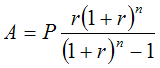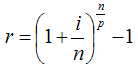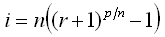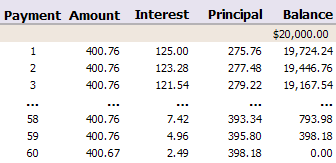The formulas used for amortization calculation can be kind of confusing. So, let's first start by describing amortization, in simple terms, as the process of reducing the value of an asset or the balance of a loan by a periodic amount . Each time you make a payment on a loan you pay some interest along with a part of the principal. The principal is the original loan amount, or the balance that you must pay off. By making regular periodic payments, the principal gradually decreases, and when it reaches zero, you've completely paid off your debt.

## Amortization Calculation

Usually, whether you can afford a loan depends on whether you can afford the periodic payment (commonly a monthly payment period). So, the most important amortization formula is the calculation of the payment amount per period.

### Calculating the Payment Amount per Period

The formula for calculating the payment amount is shown below.where
• A = payment Amount per period
• P = initial Principal (loan amount)
• r = interest rate per period
• n = total number of payments or periods

Example: What would the monthly payment be on a 5-year, \$20,000 car loan with a nominal 7.5% annual interest rate? We'll assume that the original price was \$21,000 and that you've made a \$1,000 down payment.

You can use the amortization calculator below to determine that the Payment Amount (A) is \$400.76 per month.

P = \$20,000
r = 7.5% per year / 12 months = 0.625% per period (this is entered as 0.00625 in the calculator)
n = 5 years * 12 months = 60 total periods

### Amortization Payment Calculator

 Loan Amount, P Interest Rate, r 0.01 = 1% Number of Payments, n Payment Amount, A

### Calculating the Monthly Payment in Excel

Microsoft Excel has a number of built-in functions for amortization formulas. The function corresponding to the formula above is the PMT function. In Excel, you could calculate the monthly payment using the following formula:

`=PMT(r, n, P)`
or
`=PMT(0.075/12, 5*12, 20000)`

### Calculating the Rate Per Period

When the number of compounding periods matches the number of payment periods, the rate per period (r) is easy to calculate. Like the above example, it is just the nominal annual rate divided by the periods per year. However, what do you do if you have a Canadian mortage and the compounding period is semi-annual, but you are making monthly payments? In that case, you can use the following formula, derived from the compound interest formula.where
• r = rate per payment period
• i = nominal annual interest rate
• n = number of compounding periods per year
• p = number of payment periods per year

Example: If the nominal annual interest rate is i = 7.5%, and the interest is compounded semi-annually ( n = 2 ), and payments are made monthly ( p = 12 ), then the rate per period will be r = 0.6155%.

Important: If the compound period is shorter than the payment period, using this formula results in negative amortization (paying interest on interest). See my article, "negative amortization" for more information.

If you are trying to solve for the annual interest rate, a little algebra gives:Example: Using the RATE() formula in Excel, the rate per period (r) for a Canadian mortgage (compounded semi-annually) of \$100,000 with a monthly payment of \$584.45 amortized over 25 years is 0.41647% calculated using r=RATE(25*12,-584.45,100000). The annual rate is calculated to be 5.05% using the formula i=2*((0.0041647+1)^(12/2)-1).

## Calculations in an Amortization Schedule

When you know the payment amount, it is pretty straight forward to create an amortization schedule. The example below shows the first 3 and last 3 payments for the above example. Each line shows the total payment amount as well as how much interest and principal you are paying. Notice how much more interest you pay in the beginning than at the end of the loan!The Interest portion of the payment is calculated as the rate (r) times the previous balance, and is usually rounded to the nearest cent. The Principal portion of the payment is calculated as Amount - Interest. The new Balance is calculated by subtracting the Principal from the previous balance. The last payment amount may need to be adjusted (as in the table above) to account for the rounding.

An amortization schedule normally will show you how much interest and principal you are paying each period, and usually an amortization calculator will also calculate the total interest paid over the life of the loan. Besides considering the monthly payment, you should consider the term of the loan (the number of years required to pay it off if you make regular payments). The longer you stretch out the loan, the more interest you'll end up paying in the end. Usually you must make a trade-off between the monthly payment and the total amount of interest.

To quickly create your own amortization schedule and see how the interest rate, payment period, and length of the loan affect the amount of interest that you pay, check out some of the amortization calculators listed below.

### A Note about Amortization in the UK

Some loans in the UK use an annual interest accrual period (i.e. annual compounding), but a monthly payment is calculated by dividing the annual payment by 12 and the interest portion of the payment is recalculated only at the start of each year. For these types of loans, if you create an amortization schedule using the technique described above, the schedule would need to show yearly payments (even though payments may actually be paid monthly or biweekly). For a 30-year loan at 6% you would set r = 0.06, n = 30, and p = 1 to calculate the annual payment.

### References

•  "Definition of Amortization", https://www.answers.com/amortization
• Amortization Calculator, From Wikipedia.com.